Precalculus : Find the Foci of a Hyperbola

Example Questions

2 Next →

Example Question #15 : Hyperbolas

Find the foci of the hyperbola with the following equation: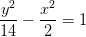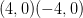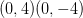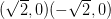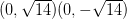Explanation:

Recall that the standard formula of a hyperbola can come in two forms: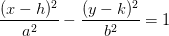and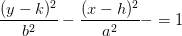, where the centers of both hyperbolas are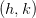.

When the term withis first, that means the foci will lie on a horizontal transverse axis.

When the term with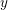is first, that means the foci will lie on a vertical transverse axis.

To find the foci, we use the following: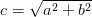For a hyperbola with a horizontal transverse access, the foci will be located at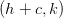and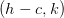.

For a hyperbola with a vertical transverse access, the foci will be located at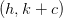and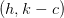.

For the given hypebola in the question, the transverse axis is vertical and its center is located at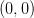.

Next, find.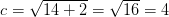The foci are then located at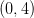and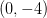.

Example Question #16 : Hyperbolas

Find the foci for the hyperbola with the following equation: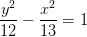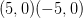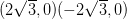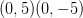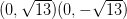Explanation:

Recall that the standard formula of a hyperbola can come in two forms:and, where the centers of both hyperbolas are.

When the term withis first, that means the foci will lie on a horizontal transverse axis.

When the term withis first, that means the foci will lie on a vertical transverse axis.

To find the foci, we use the following:For a hyperbola with a horizontal transverse access, the foci will be located atand.

For a hyperbola with a vertical transverse access, the foci will be located atand.

For the given hypebola in the question, the transverse axis is vertical and its center is located at.

Next, find.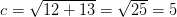The foci are then located at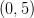and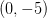.

Example Question #121 : Conic Sections

Which point is one of the foci of the hyperbola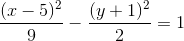?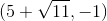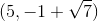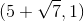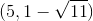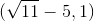Explanation:

To find the foci of a hyperbola, first determine a and b, and then use the relationship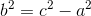In this case, the major axis is horizontal since x comes first, so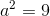and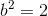.

Solve for c: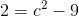add 9 to both sides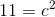take the square root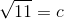Since the center is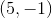and the major axis is the horizontal one, our foci are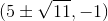. The only choice that works is.

Example Question #11 : Hyperbolas

Determine the length of the foci for the following hyperbola equation: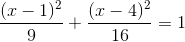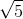Explanation:To solve, simply use the follow equation where c is the length of the foci.

In this particular case,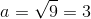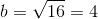Thus,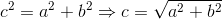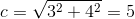Example Question #21 : Hyperbolas

Find the foci of the hyperbola with the following equation: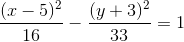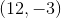and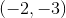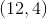and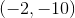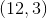and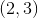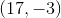and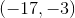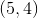and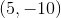andExplanation:

The standard form of the equation for a hyperbola is given by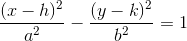The foci are located at (h+c, k) and (h-c, k), where c is found by using the formula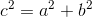Since our equation is already in standard form, you can see that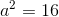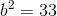Plugging into the formula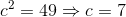So the foci are found at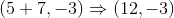AND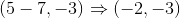2 Next →

All Precalculus Resources